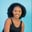Related Tags

python
communitycreator

# How to use the Numpy.square() in PythonMaria Elijah

## Overview

NumPy is a Python library that has various functions to perform calculations on the arrays of numeric data. One of these is the square() function.

### The numpy.square() function

The numpy.square() function computes the square value of each array element.

### Syntax

numpy.square(arr)


### Parameter

• arr: This represents the input array whose element we need to square.

### Code

The following code shows how to use the numpy.square() in Python:

# import numpy
import numpy as np
# create a list
my_list = [24,8,3,4,34,8]
# convert the list to numpy array
np_list = np.array(my_list)
# compute the square of each element and store it
np_list_square = np.square(np_list)

print(np_list_square)

### Explanation

• Line 2: We import the numpy library.
• Line 4: We create a list called my_list.
• Line 6: We convert the list to a NumPy array and store it in a variable np_list.
• Line 8: We use the np.square() to compute the square of each element in the np_list.
• Line 10: We display the result.

RELATED TAGS

python
communitycreator

CONTRIBUTORMaria Elijah
RELATED COURSES

View all Courses

Keep Exploring

Learn in-demand tech skills in half the time